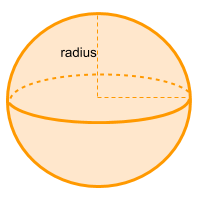Home » Non-polyhedra » Sphere

# Volume of a SphereVolume =

## Volume calculator for a sphere

Enter the radius of the sphere and get the volume automatically

## Description, how many faces, edges and vertices are there in a sphere

The sphere has a perfectly symmetrical shape, has only one curved face, no edges nor vertices and from any point on the surface to the center you get exactly the same length, in other words the radii is the same from anywhere on the surface.

## Examples of a sphere

We can find many objects that look like a sphere, examples of sphere shaped objects are: soccer and other sports balls, marbles, bowling balls and in nature bubbles or drops of water. Can you think of any other examples? leave a comment in the comment section at the bottom of the page.

## Formula for the volume of a sphere

In order to calculate the volume of a sphere the only data needed is the radius, since the symbol π is Pi and is a constant equivalent to ~ 3.14. You can also use the online calculator to automatically get the volume of the sphere .

Volume =